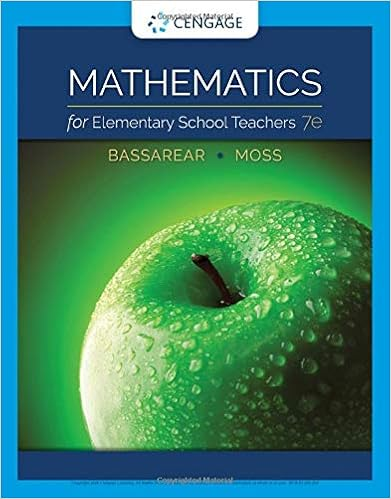# Lesson 1 MATH13-1 - Lesson 1.1 POLYGON Lesson 1.2 TRIANGLES...

• Notes
• 51

This preview shows page 1 - 14 out of 51 pages.

##### We have textbook solutions for you!
The document you are viewing contains questions related to this textbook.The document you are viewing contains questions related to this textbook.
Chapter 8 / Exercise 8
Mathematics for Elementary School Teachers
BassarearExpert Verified
Lesson 1.1: POLYGONLesson 1.2 TRIANGLESLesson 1.3 QUADRILATERALSWeek 1 and Week 2MATH13-1Solid Mensuration
##### We have textbook solutions for you!
The document you are viewing contains questions related to this textbook.The document you are viewing contains questions related to this textbook.
Chapter 8 / Exercise 8
Mathematics for Elementary School Teachers
BassarearExpert Verified
A polygonis a closed plane figure that is joined by line segments. A polygon may also be defined as a union of line segments such that: i) each endpoint is 1.1 POLYGON
Reference: Solid Mensuration by Richard Earnhart
PARTS OF A POLYGONSide or EdgeExterior AngleVertexDiagonalInterior AngleReference: Solid Mensuration by Richard Earnhart
TYPES OF POLYGONRegular PolygonIn a regular polygon, all angles are equal and all sides are of the same length. Regular polygons are both equiangular and equilateral.Equiangular PolygonA polygon is equiangular if all of its angles are congruent.Equilateral PolygonA polygon is equilateral if all of its sides are equal.Irregular PolygonA polygon that is neither equiangular nor equilateral is said to be an irregular polygon.Reference: Solid Mensuration by Richard Earnhart
NAMING OF POLYGONReference: Solid Mensuration by Richard Earnhart
Reference: Solid Mensuration by Richard EarnhartNAMING OF POLYGON
Reference: Solid Mensuration by Richard EarnhartNAMING OF POLYGON
NAMING OF POLYGONFor numbers from 100 to 999, we construct the name of the polygon by starting with the prefix for the hundreds digit taken from the ones digit minus the “gon” followed by "hecta," then proceed as before.Reference: Solid Mensuration by Richard Earnhart
Reference: Solid Mensuration by Richard EarnhartEXAMPLES
We say that two polygons are similarif their corresponding interior angles are congruent and their corresponding sides are proportional.By ratio and proportion,SIMILAR POLYGONSReference: Solid Mensuration by Richard Earnhart
.
Reference: Solid Mensuration by Richard EarnhartPROPERTIES OF A REGULAR POLYGON
Perimeter: Central Angle: Apothem: number of sides Reference: Solid Mensuration by Richard EarnhartPROPERTIES OF A REGULAR POLYGON
Area:Number of Diagonals:Interior Angle:Sum of Interior Angle:PROPERTIES OF A REGULAR POLYGONReference: Solid Mensuration by Richard Earnhart
•••